# How to Use Excel SolverClaudia Buckley#### Join the Excel conversation on Slack

Ask a question or join the conversation for all things Excel on our Slack channel.

Solver is an Excel solution used for What-if analysis. Excel Solver is similar to Goal Seek in that it works backward to achieve a numeric objective by changing variables.

Its capabilities exceed those of Goal Seek, however. Solver allows you to use up to 200 variable cells to find an optimal value for the objective cell, as opposed to Goal Seek’s one-variable-at-a-time limitation.

Additionally, Solver’s “Constraints” parameter, adds to the tool’s sophistication, because you get to tell Excel what it is and is not allowed to do in solving for the optimal value.

Solver is an add-in that needs to be enabled within the application before you can start working with it.

## How to add Solver in Excel

If the Solver add-in is already activated, you’ll see it within the Analyze group of the Data tab.If you don’t see it, do the following:

1. On the File tab, click Options.

2. Under Add-ins, select Solver Add-in and click on the Go button.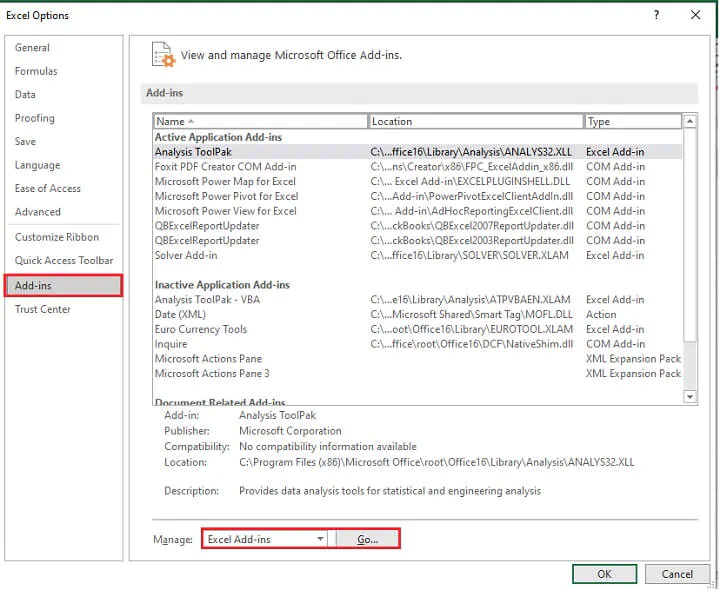3. Check Solver add-in and click OK.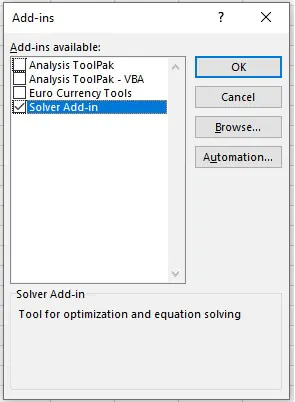The Solver command will now show up on the Data tab.

Use this free Excel Solver file to practice along with the tutorial.

## How to add Solver in Excel (Mac)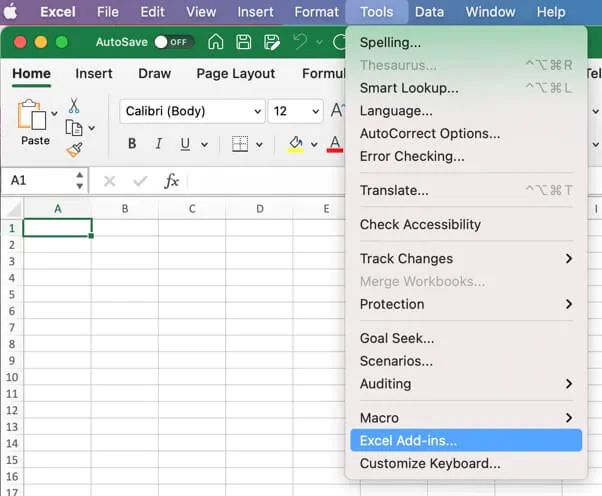1. In the Add-Ins Available box, select the Solver Add-In check box. Click OK.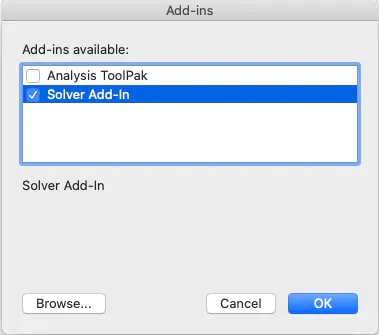1. If Solver Add-In is not listed in the Add-Ins available box, click the Browse command to find it.

Note:

• If you get a prompt that the Solver Add-In is not currently installed on your computer, click Yes to install it, then quit Excel and restart.

## How to use Solver - a case study

Solver works with variable cells to arrive at an objective, or target, subject to certain constraints. It uses the variable cells to produce the result you want in the objective cell. You can see from this that:

1. The objective cell must contain a formula.
2. The formula in the objective cell must be directly or indirectly dependent on the variable cells.

In our example, we run an outsourcing company that handles short-term projects for our clients. Clients give us their project objectives, scope, and deadlines and that helps us to know how many agent hours will be needed that week.

The Workforce Management (Agent Scheduling) team can use Solver to create weekly employee schedules to satisfy these objectives within whatever constraints we may have. Some possible constraints are:

• The minimum and/or maximum number of hours each employee may be scheduled daily.
• The minimum and/or maximum number of hours each employee may be scheduled weekly.
• The maximum number of overtime hours we want to schedule (i.e., wage cost factor).

Before we can begin to think about the constraints, however, what is our objective? We want to dedicate our employee resources to a certain number of hours to work on our available projects for the upcoming week.

Let’s assume that we have three weeks in which to complete these projects and 10 employees. All we need to do is spread the 1,065 hours across three weeks and distribute those hours to the 10 employees.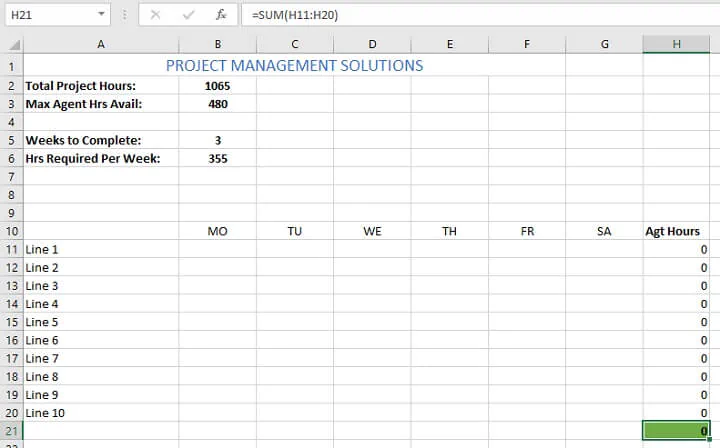Cells H11 to H20 are set to calculate the number of agent hours in each line.

Cell H21 is set to calculate the total number of hours for all agents.

As the Workforce Manager, you may want to experiment with a few scenarios, such as:

1. Spreading the hours evenly across three weeks.
2. Maximizing agent hours this week (maybe you expect additional projects in the upcoming weeks and want to get these done as quickly as possible).
3. Scheduling the maximum allowable number of employee hours without incurring overtime, and so on.

To make the cell ranges easier to work with, we’ve created named ranges as follows:

• Agt_Hrs (cells H11:H20), and
• Shifts (cell B11:G20).

## Formulate the model

To get started, click on the Solver command, which opens up the Solver Parameters dialog box.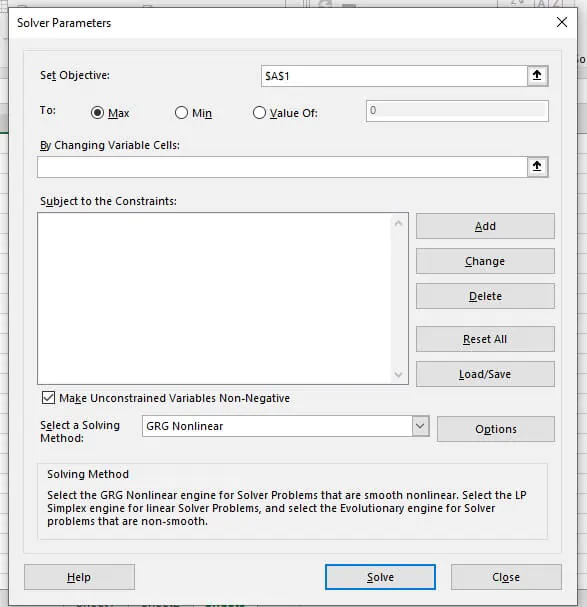Let’s define the first three terms in this window.

 Set Objective: This field refers to the cell which will contain a single, quantifiable result. Decide whether you want to solve for the minimum, maximum, or for an exact value. These are cells that Excel can change to achieve the objective. Excel Solver allows specifying up to 200 variable cells.

Now we can use the above fields to set up our problem and its solution. Our Set Objective: cell will be cell H21. Remember, this cell calculates the total number of scheduled working hours for all agents next week.

Let’s consider spreading the 1,065 hours required across approximately three weeks. This would mean scheduling 355 hours each week. Select the Value of: radio button and enter 355.

By Changing Variable cells: will be the range that we named Shifts.

### Constraints

Now we can move on to the constraints — that is, what Excel is and isn’t allowed to do when trying to solve the problem. Constraints in Solver fall within the realm of:

 >= The value in this cell must be greater than or equal to …. The value in this cell must be less than or equal to …. The value in this cell must be equal to …. This variable must be an integer The value of this variable is binary (must be either 0 or 1) The variables in this range must be all different. No repetition is allowed.

In our case study example, we want to achieve our 355 hours without anyone having to work overtime (more than 40 hours). This is achievable since we have 10 agents. Maybe there’s a union regulation that says all employees are guaranteed a minimum of 20 hours per week. We can add these constraints by clicking Add to the right of the Solver Parameters window.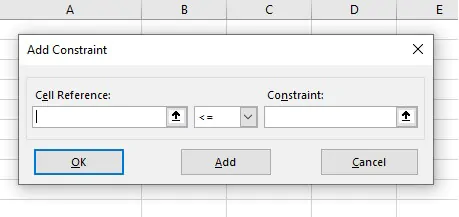Agt_Hrs>=20

Agt_Hrs<=40

Click OK to submit these constraints and return to the Solver Parameters dialog box.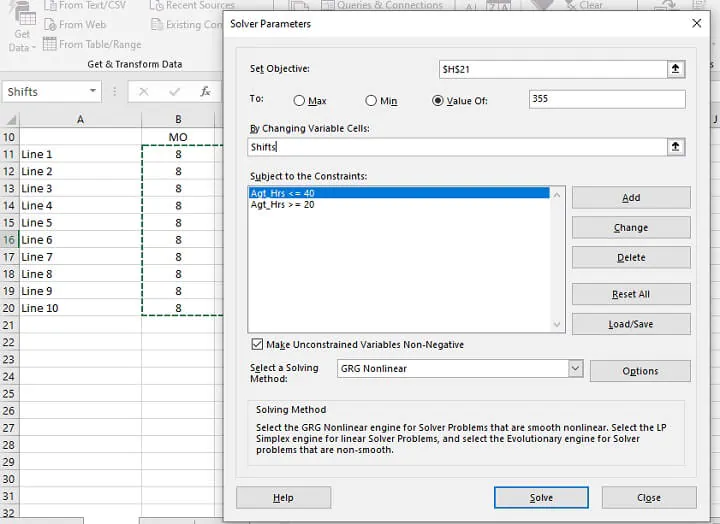We have a few more constraints to enter. These relate to the shifts being scheduled.

1. Employee shifts should be 8 hours or less (Shifts <=8).
2. Employee shifts should be whole numbers (Shifts = integer).
3. We also want our Objective cell to be equal to the value in B6 (H21 = B6).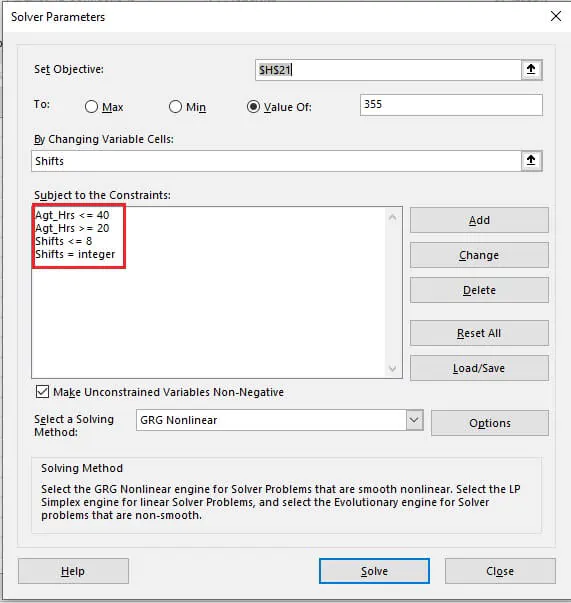### Edit a constraint

You can edit a constraint by clicking on it, then selecting Change on the right of the Solver Parameters window.

You can delete a constraint by clicking it, then selecting Delete on the right of the Solver Parameters window.

### Solving methods (Excel Solver algorithms)

You’ll notice the following options for your solving methods:

• Simplex LP

This method is used for linear problems. A Solver model is considered linear when the Objective cell is computed by adding together the terms of the (variable cells)*(constant) form. In linear problems, each constraint is also evaluated by adding together the terms of the (variable cells)*(constant) form and comparing the sums to a constant. When the Simplex LP Solving Method is selected, there is no limit on the number of constraints.

• Generalized Reduced Gradient (GRG) Nonlinear

The GRG Nonlinear method is used for smooth nonlinear problems. If your target cell, any of your constraints, or both, contain references to changing cells that are not of the (variable cells)*(constant) form, then you have a nonlinear model.

When the GRG Nonlinear Evolutionary Solving Method is selected, there is a limit of 100 constraints, in addition to bounds and int, bin or dif restrictions on the variables.

• Evolutionary

The Evolutionary solving method is used for non-smooth problems. These are the most difficult types of optimization problems to solve because some of the functions are non-smooth or even discontinuous.

We will choose the LP Simplex method for our model since this is a linear problem. If you are not sure of your problem type, you can leave it at GRG Nonlinear.

## Solve!

Now we can set our Solver to work. Since we have a constraint that says that the objective cell should be equal to the value in cell B6, it will try to meet that goal whether we use the Min or Max radio button.

The optimal solution is shown below.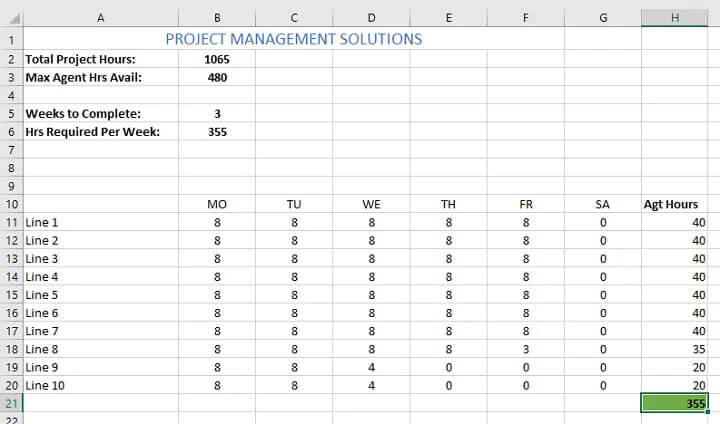Excel finds a solution that meets all constraints by plotting the number of hours agents should be scheduled to work in the upcoming week.

## How to save an Excel Solver scenario

When Excel provides a solution, you may want to save your scenario and experiment with a few other options before deciding on which one to use.

1. In the Solver Parameters window, click the Load/Save button.
2. Excel Solver will tell you how many cells are needed to save your scenario. Select that number of empty cells and click Save.
3. Excel will save your current model, which may look something like this: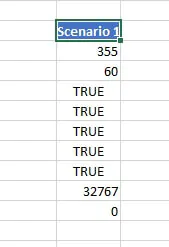At the same time, the Solver Parameters window will show up where you can change your constraints and try different "what if" options.

## How to load a saved model

To restore a saved scenario, do the following:

1. In the Solver Parameters window, click the Load/Save button.
2. On the worksheet, select the range of cells holding the saved model and click Load.
1. In the “Load Model” dialog box, click the Replace button.
2. This will open the main Excel Solver window with the parameters of the previously saved model. Click the Solve button to recalculate it.

Use this free Excel Solver file to practice along with the tutorial.

Still getting the hang of Solver? Practice makes perfect! Experiment with different scenarios, and save them if you like the results or want to return to them later.

• See what happens if you remove the H21 = B6 constraint. If the radio button remains at Max, then Solver will find the optimal solution with the maximum allowed by the defined constraints.
• Or set the objective to a specific value, say 400, and see how that changes your model.

## Everyday uses

Solver can be applied to workforce management, maximizing profitability, financial planning, and budgeting settings. Can you think of any other ways you can find optimal solutions for some of your problems using the Solver add-in?

Explore other useful Excel tools with our Excel Basic and Advanced course today!

Ready to become a certified Excel ninja?